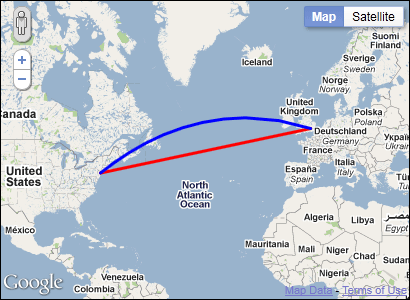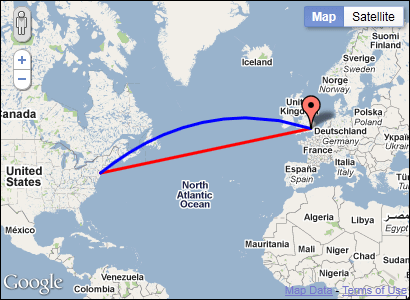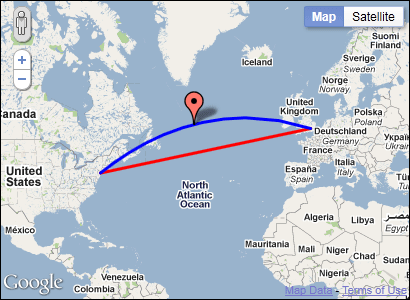In usability we trust

UX and all things webAs I mentioned in the article Extending Google Maps API 3 with libraries a couple of weeks ago, you can add functionality to the Google Maps API by using libraries. One of these libraries are the Geometry Library. In this article I will show how you can use the function of that library to calculate distances and areas. I will also explain some additional navigation functions that you might find useful.

Including the library

First of all to be able to use the library you need to let the Google Maps API know that you want to use it. This is done by adding an extra parameter to the querystring when including the Maps API on the web page.

Namespaces

Once you’ve done that you have a number of extra functions that you can use. They all reside in one of two namespaces:

The spherical namespace includes functions for calculating angles, distances and areas from latitudes and longitudes. These are the functions that we will examine in this article.

The other namespace, encoding, contains functions for encoding and decoding paths for polylines and polygons. They’re useful when you have big sets of data for displaying polylines or polygons that you need to optimize. I will not describe this namespace further here, maybe it’s a subject for a future article.

Note: The library doesn’t consist of any classes but only have static functions.

The earth is round, the map is flat

When calculating distances and areas you need to take the fact that the earth is round into consideration (hence the name spherical). Since a map is flat a straight line on it is in fact an arc. Take a look at the map below. The shortest route between New York and London will look like an arc on a flat map (the blue line in the image below).A straight line between two point on a map is in fact an arc.

Therefor when calculating distances and areas you need to use some pretty advanced mathematics to do it. Fortunately with the Geometry Library it’s dead simple.

Distances and areas

Calculating the distance

Calculating the distance between two points is really easy with the help of the Geometry library. All you have to do is to call the computeDistanceBetween() function and pass it two LatLng objects.

var nyc = new google.maps.LatLng(40.715, -74.002);
var london = new google.maps.LatLng(51.506, -0.119);

The function returns the distance in meters. In this case it says that it’s 5 576 673 meters between NYC and London.

If you also want to know the heading between two points you can use the computeHeading() function. It also takes two LatLng objects and determines the heading from the first to the second.

var nyc = new google.maps.LatLng(40.715, -74.002);
var london = new google.maps.LatLng(51.506, -0.119);

The heading is returned in degrees from the true north (counts clockwise from 0). The heading from NYC to London is 51.2145583127633 degrees.

Calculating an area

To calculate the size of an area there’s the computeArea() function. Unlike the two previous functions it takes an array of LatLng objects as a parameter. The LatLng’s in this array represents a polygon and it must be closed, meaning that it will automatically close the polygon by connection the first and the last LatLng.

Note: You can also pass a MVCArray as a parameter. It’s basically a regular array but is API specific and has a few other advantages.

var nyc = new google.maps.LatLng(40.715, -74.002);
var london = new google.maps.LatLng(51.506, -0.119);
var rio = new google.maps.LatLng(-22.916, -43.251);
var area = google.maps.geometry.spherical.computeArea([nyc, london, rio]);

The code above will calculate the area of a triangle between NYC, London and Rio de Janeiro which is 26 566 710 985 447.367 m2

There are a few additional functions apart from the ones that calculates distances and areas.

Calculating an end location

If for example, you know the starting point, the distance and the heading. You can get the end location by using the computeOffset() function. It takes the starting point, the distance and the heading as parameters and returns a LatLng object.

If we take the values from the previous examples, the location of NYC, the distance to London and the heading towards London and use them as input.

var nyc = new google.maps.LatLng(40.715, -74.002);
var distance = 5576673;
position: endPoint,
map: map
});

In this case endPoint will be the location of London which is LatLng(51.506, -0.118). (it differs slightly because I’ve rounded the numbers)With the computeOffset() function we can get the end location if we know the starting point, the heading and the distance.

Calculate a point in between

Another useful function is interpolate() which determines a point between two locations based on a fraction between 0 and 1. The fraction represents how far between two locations a point is. So for example 0.5 is a point right in the middle between the start- and end location.

var nyc = new google.maps.LatLng(40.715, -74.002);
var london = new google.maps.LatLng(51.506, -0.119);
var inBetween = google.maps.geometry.spherical.interpolate(nyc, london, 0.5);
position: inBetween,
map: map
});

This code will return a position right between NYC and London.By using interpolate between NYC and London with the fraction 0.5 we get the position right in the middle of those.

Conclusion

Calculating areas and distances on geo data can be tricky, but with the help of the Geometry Library it’s really simple. I hope this article has shed some light on how you can use it in your own map solution.

More resources

To read more about this library, check out the article Google Maps Javascript API V3 Geometry Library in the offical documentation. You’ll also find a description of all the functions in the API documentation.

1.Hi,

should be

2.rca: Good catch! Thanks for letting me know. I’ve updated the article so that it’s now correct!

3.question I find that 3.2 api does not have the geomentry function google.maps.geometry.spherical.computeHeading. Looks like it has 4 values you can take 0, 90, 180, 270. Is there any other way to compute heading in 3.2

4.Selvi: There’s no other way of computing headings in v 3.2 that I’m aware of. But you could of course do the calculations yourself.

5.6.Great tutorial.
Is there any php library with functions like google.maps.geometry.spherical.computeOffset ?

7.Gabriel,
Post was of great help. I trying to draw the arc between 2 points, say a flight plan between nyc and london (the blue line above). How would you do it ? I could use the polyline encoding utility provided by Google and manually to try to get an arc. Is there a simpler method ?
Thanks
Sriram

•@Buytowels: It’s quite simple really. You just create a regular Polyline but add the option geodesic: true. The docs say that this option:

“Render each edge as a geodesic (a segment of a “great circle”). A geodesic is the shortest path between two points along the surface of the Earth.”

•@Buytowels: It’s quite simple really. You just create a regular Polyline but add the option geodesic: true. The docs say that this option:

“Render each edge as a geodesic (a segment of a “great circle”). A geodesic is the shortest path between two points along the surface of the Earth.”

8.ah, what an awesome guide! has really helped me a lot with my current project 🙂

9.Awesome mate!

10.incredibly objective and useful. saved me at least 6 hours converting my android javascript code (no convenient libraries that I know of). thanx a zillion!!!!

11.hi, i have one question, can i in some way, check if an address belongs into a quadrant defined by a geometric using gmaps?

12.How can I get the midpoint on red line (straight line between NYC & London ) ?

13.hi,
i want to use geometry librarie, for this i follow this step:
first i Including the library:

second i try to calculate distance: var nyc = new google.maps.LatLng(40.715, -74.002);
var london = new google.maps.LatLng(51.506, -0.119);

this is all my code(index.html):

but he shows: Le serveur API Google Maps a rejeté votre demande. Le paramètre “sensor” indiqué doit être défini sur “true” ou “false”

i try to change the parametre “sensor” by true or false only but i got not the distance.

what is the problem
help

14.Christian Franco

August 16, 2012 at 3:12 pm

Thanks a lot

15.Does anybody can help me to make 3 point great circle pat in google maps!

I have made javascript for showing great circle diatance between 2 points and seems I have problem with hree dimensional Array,..I’m stucked :-(,..any hints guys ??

Tim

16.Very nice article , helped me alot, thank you Gabriel!

•No problems! Thanks for the feedback!

•hi I am getting Unable to get value of the property ‘spherical’: object is null or undefined…..i have added the api3 also not sure why….what I need to do…I added like this var

•my bad forgot to include lib…..can u tell me what sensor do if i gave true or false what happen ?
sensor=true_or_false
ans also thanks for sharing nice article

17.Elizabeth Ferguson

April 8, 2018 at 5:15 pm

Thank you for the great guide!